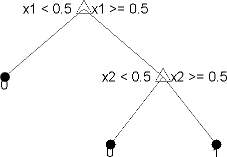# Accuracy of each path in a decision tree in Matlab

I would like to know the accuracy of each path in a decision tree in Matlab.

I can build a decision tree in Matlab by:

ctree = ClassificationTree.fit(X,Y)


Where X is a matrix of instances (rows are observations, columns are variables), and Y is the response variable.

Here's an example of a simple output tree:For example, the above tree has three different paths:

1. (x1 < .5) => class 0

2. (x1 >= .5) And (x2 < .5) => class 0

3. (x1 >= .5) And (x2 >= .5) => class 1

Now I would like to get the accuracy/confidence of each path. Is there a way to do that?

For example, I want to know how many instances were classified as 0 through the first path and they were actually class 0 (I can provide the same training set X,Y for that)

If I use the function resubLoss:

resuberror = resubLoss(ctree)


I will get the overall accuracy by resubmitting the same training set.

I'm confused why I could not find what I'm looking for, it seems that it's super useful/important.

If you use [yfit,nodes] = eval(ctree,X); nodes will contain the node number assigned to each row of X. Since each leaf has a unique path to root, you'll get what you're looking for using nodes.